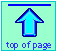Note: this document will print in an appropriately modified format (8 pages)

1  What is energy?

Energy is one of the most fundamental and universal concepts of physical science, but one that is remarkably difficult to define in way that is meaningful to most people. This perhaps reflects the fact that energy is not a “thing” that exists by itself, but is rather an attribute of matter (and also of electromagnetic radiation) that can manifest itself in different ways. It can be observed and measured only indirectly through its effects on matter that acquires, loses, or possesses it.

The concept that we call energy was very slow to develop; it took more than a hundred years just to get people to agree on the definitions of many of the terms we use to describe energy and the interconversion between its various forms. But even now, most people have some difficulty in explaining what it is; somehow, the definition we all learned in elementary science ("the capacity to do work") seems less than adequate to convey its meaning.

Although the term "energy" was not used in science prior to 1802, it had long been suggested that certain properties related to the motions of objects exhibit an endurance which is incorporated into the modern concept of "conservation of energy". René Descartes (1596-1650) stated it explicitly:

When God created the world,  He "caused some of its parts to push others and to transfer their motions to others..." and thus "He conserves motion".*

In the 17th Century, the great mathematician Gottfried Leibniz (1646-1716) suggested the distinction between vis viva ("live force") and vis mortua ("dead force"), which later became known as kinetic energy (1829) and potential energy (1853).

### Kinetic energy and potential energy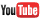A Mark Rosengarten video on potential and kinetic energy
(9 min)

Whatever energy may be, there are basically two kinds.

Kinetic energy is associated with the motion of an object, and its direct consequences are part of everyone's daily experience; the faster the ball you catch in your hand, and the heavier it is, the more you feel it.  Quantitatively, a body with a mass m and moving at a velocity v  possesses the kinetic energy mv2/2.

Potential energy is energy a body has by virtue of its location. But there is more: the body must be subject to a "restoring force" of some kind that tends to move it to a location of lower potential energy. Think of an arrow that is subjected to the force from a stretched bowstring; the more tightly the arrow is pulled back against the string, the more  potential energy it has.

More generally, the restoring force comes from what we call a force field— a gravitational, electrostaticl, or magnetic field. We observe the consequences of gravitational potential energy all the time, such as when we walk, but seldom give it any thought.

If an object of mass m is raised off the floor to a height h, its potential energy increases by mgh, where g is a proportionality constant known as the acceleration of gravity; its value at the earth's surface is 9.8 m s–2.

Similarly, the potential energy of a particle having an electric charge q depends on its location in an electrostatic field.

### "Chemical energy"

Electrostatic potential energy plays a major role in chemistry; the potential energies of electrons in the force field created by atomic nuclei lie at the heart of the chemical behavior of atoms and molecules.

"Chemical energy" usually refers to the energy that is stored in the chemical bonds of molecules. These bonds form when electrons are able to respond to the force fields created by two or more atomic nuclei, so they can be regarded as manifestations of electrostatic potential energy.

In an exothermic chemical reaction, the electrons and nuclei within the reactants undergo rearrangment into products possessing lower energies, and the difference is released to the environment in the form of heat.

### Interconversion of potential and kinetic energy

Transitions between potential and kinetic energy are such an intimate part of our daily lives that we hardly give them a thought. It happens in walking as the body moves up and down.Our bodies utilize the chemical energy in glucose to keep us warm and to move our muscles. In fact, life itself depends on the conversion of chemical energy to other forms.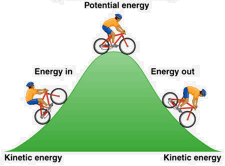Energy is conserved: it can neither be created nor destroyed. So when you go uphill, your kinetic energy is transformed into potential energy, which gets changed back into kinetic energy as you coast down the other side. And where did the kinetic energy you expended in peddling uphill come from? By conversion of some of the chemical potential energy in your breakfast cereal.

• When you drop a book onto the floor, its potential energy is transformed into kinetic energy. When it strikes the floor, this transformation is complete. What happens to the energy then? The kinetic energy that at the moment of impact was formerly situated exclusively in the moving book, now becomes shared between the book and the floor, and in the form of randomized thermal motions of the molecular units of which they are made; we can observe this effect as a rise in temperature.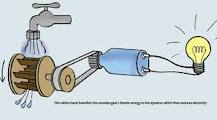[image]

• ← Much of the potential energy of falling water can be captured by a water wheel or other device that transforms the kinetic energy of the exit water into kinetic energy. The output of a hydroelectric power is directly proportional to its height above the level of the generator turbines in the valley below. At this point, the kinetic energy of the exit water is transferred to that of the turbine, most of which (up to 90 percent in the largest installations) is then converted into electrical energy.
• Will the temperature of the water at the bottom of a water fall be greater than that at the top? James Joule himself predicted that it would be. It has been calculated that at Niagra falls, that complete conversion of the potential energy of 1 kg of water at the top into kinetic energy when it hits the plunge pool 58 meters below will result in a temperature increase of about 0.14 C°. (But there are lots of complications. For example, some of the water breaks up into tiny droplets as it falls, and water evaporates from droplets quite rapidly, producing a cooling effect.)
•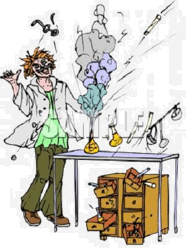Chemical energy can also be converted, at least partially, into electrical energy: this is what happens in a battery.  If a highly exothermic reaction also produces gaseous products, the latter may expand so rapidly that the result is an explosion — a net conversion of chemical energy into kinetic energy (including sound). [image]

### Thermal energy

Kinetic energy is associated with motion, but in two different ways.  For a macroscopic object such as a book or a ball, or a parcel of flowing water, it is simply given by ½ mv2.

But as we mentioned above, when an object is dropped onto the floor, or when an exothermic chemical reaction heats surrounding matter, the kinetic energy gets dispersed into the molecular units in the environment.  This "microscopic" form of kinetic energy, unlike that of a speeding bullet, is completely random in the kinds of motions it exhibits and in its direction. We refer to this as "thermalized" kinetic energy, or more commonly simply as thermal energy. We observe the effects of this as a rise in the temperature of the surroundings. The temperature of a body is direct measure of the quantity of thermal energy is contains.

#### Convsersion of thermal energy to heat is never completely recoverable

Once kinetic energy is thermalized, only a portion of it can be converted back into potential energy.  The remainder simply gets dispersed and diluted into the environment, and is effectively lost.

To summarize, then:

• Potential energy can be converted entirely into kinetic energy..
• Potential energy can also be converted, with varying degrees of efficiency,into electrical energy.
• The kinetic energy of macroscopic objects can be transferred between objects (barring the effects of friction).
• Once kinetic energy becomes thermalized, only a portion of it can be converted back into either potential energy or be concentrated back into the kinetic energy of a macroscopic. This limitation, which has nothing to do with technology but is a fundamental property of nature, is the subject of the second law of thermodynamics.
• A device that is intended to accomplish the partial transformation of thermal energy into organized kinetic energy is known as a heat engine.

#### Some examples of potential-kinetic energy interconversion processes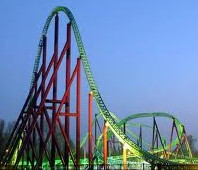Roller coasterElastic potential energyGoing downhillBungee bouncing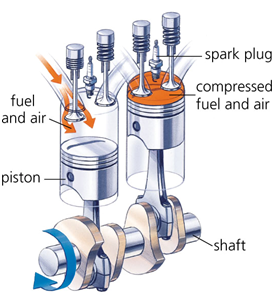4-stroke gas engine Click image to enlargePendulumFireworks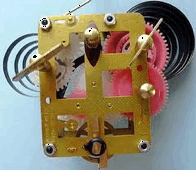Wind-up clock)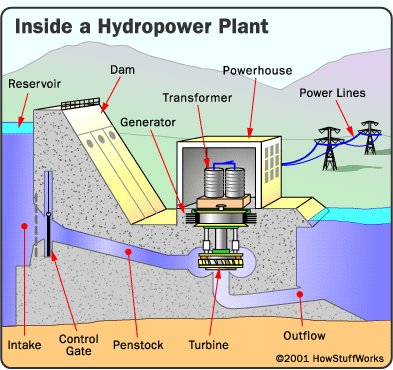Schematic diagram of a
large hydroelectric plant [more...]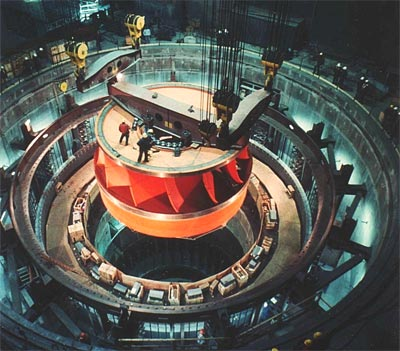Turbine being installed at Grand Coulee dam, 1974.

Hydroelectric power constitutes one of the major engineering accomplishments that exploits conversion of potential energy into kinetic– and thence into electrical energy.

← Click on these images to enlarge them.

2  Energy scales and units

### Energy scales are always arbitrary

You might at first think that a book sitting on the table has zero kinetic energy since it is not moving. But if you think about it, the earth itself is moving; it is spinning on its axis, it is orbiting the sun, and the sun itself is moving away from the other stars in the general expansion of the universe. Since these motions are normally of no interest to us, we are free to adopt an arbitrary scale in which the velocity of the book is measured with respect to the table; on this so-called laboratory coordinate system, the kinetic energy of the book can be considered zero.

We do the same thing with potential energy. If the book is on the table, its potential energy with respect to the surface of the table will be zero. If we adopt this as our zero of potential energy, and then push the book off the table, its potential energy will be negative after it reaches the floor.

### Energy units in chemistry and engineering

Energy is measured in terms of its ability to perform work or to transfer heat. Mechanical work is done when a force f displaces an object by a distance d:
w = f × d. The basic unit of energy is the joule. One joule is the amount of work done when a force of 1 newton acts over a distance of 1 m; thus 1 J = 1 N-m. The newton is the amount of force required to accelerate a 1-kg mass by 1 m/sec2, so the basic dimensions of the joule are kg m2 s–2. The other two units in wide use. the calorie and the BTU (British thermal unit) are defined in terms of the heating effect on water. Because of the many forms that energy can take, there are a correspondingly large number of units in which it can be expressed, a few of which are summarized below.

 1 calorie will raise the temperature of 1 g of water by 1 C°. The “dietary” calorie is actually 1 kcal. An average young adult expends about 1800 kcal per day just to stay alive. (you should know this definition) 1 cal = 4.184 J 1 BTU (British Thermal Unit) will raise the temperature of 1 lb of water by 1F°. 1 BTU = 1055 J The erg is the c.g.s. unit of energy and a very small one; the work done when a 1-dyne force acts over a distance of 1 cm. 1 J = 107 ergs 1 erg = 1 d-cm = 1 g cm2 s–2 The electron-volt is even tinier: 1 e-v is the work required to move a unit electric charge (1 C) through a potential difference of 1 volt. 1 J = 6.24 × 1018 e-v The watt is a unit of power, which measures the rate of energy flow in J sec–1. Thus the watt-hour is a unit of energy. An average human consumes energy at a rate of about 100 watts; the brain alone runs at about 5 watts. 1 J = 2.78 × 10–4 watt-hr 1 w-h = 3.6 kJ The liter-atmosphere is a variant of force-displacement work associated with volume changes in gases. 1 L-atm = 101.325 J The huge quantities of energy consumed by cities and countries are expressed in quads; the therm is a similar but smaller unit. 1 quad = 1015 Btu = 1.05 × 1018 J If the object is to obliterate cities or countries with nuclear weapons, the energy unit of choice is the ton of TNT equivalent. 1 ton of TNT = 4.184 GJ (by definition) In terms of fossil fuels, we have barrel-of-oil equivalent, cubic-meter-of-natural gas equivalent, and ton-of-coal equivalent. 1 bboe = 6.1 GJ 1 cmge = 37-39 mJ 1 toce = 29 GJ
3  Heat and work

Heat and work are both measured in energy units, so they must both represent energy. How do they differ from each other, and from just plain “energy” itself?

### Heat and work are processes and cannot be stored

In our daily language, we often say that "this object contains a lot of heat", but this kind of talk is a no-no in thermodynamics! It's ok to say that the object is "hot", meaning that its temperature is high.

The term "heat" has a special meaning in thermodynamics: it is a process in which a body (the contents of a tea kettle, for example) acquires or loses energy as a direct consequence of its having a different temperqture than its surroundings (the rest of the world).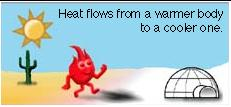Thermal energy can only flow from a higher temperature to a lower temperature. It is this flow that constitutes "heat".

Use of the term "flow" of heat recalls the 18th-century notion that heat is an actual substance called “caloric” that could flow like a liquid.

#### Heat is the transfer of energy by conduction or radiation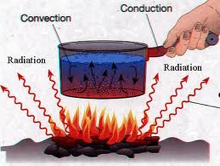Transfer of thermal energy can be accomplished by bringing two bodies into physical contact (the kettle on top of the stove, or through an electric heating element inside the kettle). Another mechanism of thermal energy transfer is by radiation; a hot object will convey energy to any body in sight of it via electromagnetic radiation in the infrared part of the spectrum. In many cases, a combination of modes will be active:

Thus when you place a can of beer in the refrigerator, both processes are operative: the can radiates heat to the cold surfaces around it, and absorbs it by direct conduction from the ambient air.

### So what is work?

Work refers to the transfer of energy some means that does not depend on temperature difference.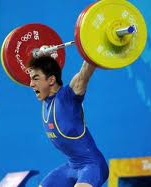Work, like energy, can take various forms, the most familiar being mechanical and electrical. Mechanical work arises when an object moves a distance Δx against an opposing force f: w = f Δx N-m; 1 N-m = 1 J.

[image from Ben Wiens Energy site]

Electrical work is done when a body having a charge q moves through a potential difference ΔV.

Work, like heat, exists only when energy is being transferred.

When two bodies are placed in thermal contact and energy flows from the warmer body to the cooler one,we call the process “heat”. A transfer of energy to or from a system by any means other than heat is called “work”.

### Heat can only partially be converted into work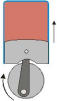Work can be completely converted into heat (by friction, for example), but heat can only be partially converted to work. Conversion of heat into work is accomplished by means of a heat engine, the most common example of which is an ordinary gasoline engine. The science of thermodynamics developed out of the need to understand the limitations of steam-driven heat engines at the beginning of the Industrial Age. A fundamental law of Nature, the Second Law of Thermodynamics, states that the complete conversion of heat into work is impossible. Something to think about when you purchase fuel for your car!